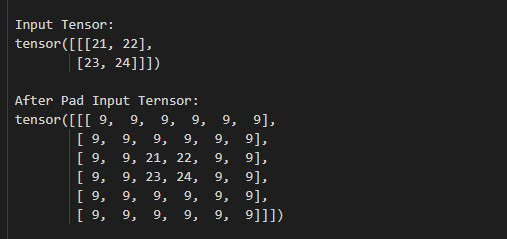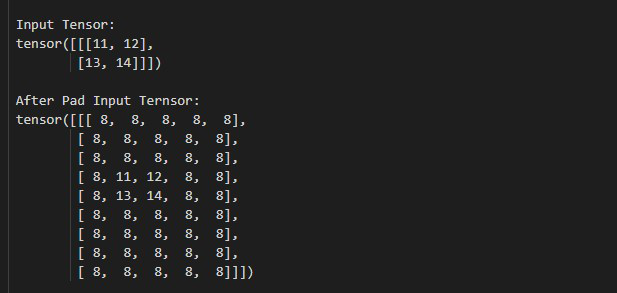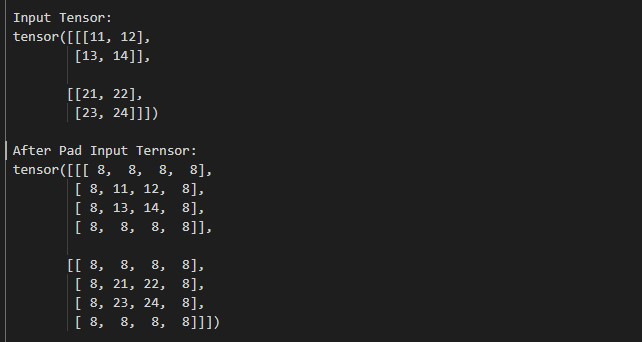Open in App
Not now

# How to Pad the Input Tensor Boundaries With a Constant Value in PyTorch?

• Last Updated : 10 Oct, 2022

In this article, we will discuss How to Pad the Input Tensor Boundaries With a Constant Value in PyTorch. We can pad the input tensor boundaries with a constant value by using torch.nn.ConstantPad2D() method.

We can pad the boundaries of 3D and 4D tensors and the shape of the input tensor is [C, H, W] and [N, C, H, W] respectively, where N represents the mini-batch size, C represents the number of channels, and  H, W represents the height and width respectively. This method accepts the size of padding and a constant value as input. The boundaries may be the same or different from all sides (left, right, top, bottom). we can increase the height and width of a padded tensor by using top+bottom and left+right respectively. The below syntax is used to pad the input tensor boundaries with a Constant Value.

Parameter:

• pad (int, tuple): This is size of padding. The size of padding is an integer or a tuple.
• value: This is constant value.

Return: This method returns a new tensor with boundaries.

### Example 1:

In this example, we will see how to add the same padding sizes to all sides.

## Python3

 `# Import required library``import` `torch``import` `torch.nn as nn` `# define a tensor``tens ``=` `torch.tensor([[[``21``, ``22``], [``23``, ``24``]]])``print``(``"\n Input Tensor: \n"``, tens)` `# give padding size same for all sides``pad ``=` `nn.ConstantPad2d(``2``, ``9``)``output ``=` `pad(tens)` `# display result``print``(``"\n After Pad Input Tensor: \n"``, output)`

Output:### Example 2:

In this example, we will see how to add unique padding sizes to all sides (left, right, top, bottom).

## Python3

 `# Import required library``import` `torch``import` `torch.nn as nn` `# define a tensor``tens ``=` `torch.tensor([[[``11``, ``12``], [``13``, ``14``]]])``print``(``"\n Input Tensor: \n"``, tens)` `# add unique padding sizes to all sides``# (left, right, top, bottom)``pad ``=` `nn.ConstantPad2d((``1``, ``2``, ``3``, ``4``), ``8``)``output ``=` `pad(tens)` `# display result``print``(``"\n After Pad Input Tensor:\n"``, output)`

Output:Example 3:

In this example, we will see how to pad the boundaries of a batch of tensors.

## Python3

 `# Import required library``import` `torch``import` `torch.nn as nn` `# define a batch of tensor``tens ``=` `torch.tensor([[[``11``, ``12``], [``13``, ``14``]],``                     ``[[``21``, ``22``], [``23``, ``24``]]])` `print``(``"\n Input Tensor: \n"``, tens)` `# add unique padding sizes to all sides``# (left, right, top, bottom)``pad ``=` `nn.ConstantPad2d(``1``, ``8``)``output ``=` `pad(tens)` `# display result``print``(``"\n After Pad Input Tensor:\n"``, output)`

Output:My Personal Notes arrow_drop_up